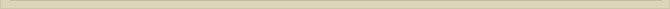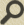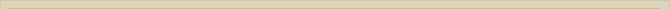## Discussion on QM and the ether

When you are interested in physics you must read “Unbelievable“!

We described the hydrogen atom as an EM-free rotator and found complete consistent formulas with QM. Scientists state that Bohr’s atom model is invalid for atoms when the charge of the nucleus exceeds the charge of the positron (Z>1) and that therefore the presented EM-free rotator for atoms when Z>1 must be invalid to.

The reason why Bohr’s atomic model is not adequate to describe atoms when Z>1 is that the formula for the (macroscopic) Coulomb force between two charges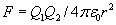is only valid in describing the electrostatic force between charges in our macro-world. The Coulomb force is an in our macro-world experimental derived formula. The QM-rules at subatomic levels are not relevant anymore in our macro world and for that reason the Coulomb-force formula is not valid at QM-levels. At the subatomic (ionization) levels there is interference of the electrostatic fields of the positive charges in the nucleus. This interference disappears in the macro-world. The Coulomb-force for the EM-free rotator and Bohr’s model should be, because of the interference of electric fields at sub-atomic levels, be :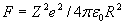or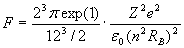(***)

Interference occurs at subatomic levels because the electrostatic fields of the protons in the nucleus seek a way out. Not all point-volumes around the nucleus are in touch (inhomogeneous space) so the resistance for electrostatic fields differs around the nucleus. The different fields of the protons in the nucleus follow the same low resistance “route” in space (=interference).

The electrostatic field around a nucleus is not homogeneous. Interference of electrostatic fields at subatomic level is expected while at our 3-dimensional world space/vacuum is homogenous.

In general QM describes mathematically the physics at molecular level and sub-atomic level very well. This is even so when one realizes that the use of mathematical correction factors QM is not uncommon. Despite the significance of the mathematical solutions QM offers there is a serious flaw; the physics behind the QM-math are not understood.

The perspective of science concerning vacuum is an absolute empty space, although in Theoretical Physics the field theory is widely accepted and contradicts at least philosophically the assumed absolutely empty space.

What is the chance that coincidence the rydberg-distance is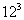time the Bohr-radius, and that the Bohr-radius istimes the Compton-radius, and that the Compton-radius istimes the Planck-radius and that at the same time the 12 atomic ionization levels of the atom are identified, Planck’s constant eliminated as an independent natural constant, the origin of the mathematical constant e and π is located and the mysterious aspects of molecular QM are answered?

These observations and the high likelihood of space not being absolutely empty, the acceptance Science of the field theory and the complete explanation of the phenomenon of stellar aberration, should be able to persuade scientists to at least look in to the matter. However new theoretical ideas and discoveries countermarches established and traditional theses and therefore are fiercely rejected science.

Is it impossible that science erroneously concluded that vacuum is absolute empty space?

Complete mathematical and physical understanding of QM in the case of Bohr’s atomic model can be achieved when we consider space filled with point-volumes with radius QD. The matrix of point-volumes filling up space around the nucleus is imperfect for electrons (Rc) at distances smaller than the rydbergconstant. The resonance ofQD in the “matrix of space” from the Planck-distance to the rydberg-distance can be simulated mathematically. This simulation will show the 12-ionization levels of the electron orbiting around the nucleus. Many, many other quantum resonance distances between the QD and the rydberg-distance will be identified.

The reader should realize that the above shown relations between Rr/Rb=Rb/Rc=Rc/QD=is the consequence of the 3-dimensional properties of the electron. The electron circles around the nucleus and the dimension of the electron determines the distances where space is “perfect” for the electron. Should the electron have other dimensions than Rc the observed distances Rr and Rb would change accordingly.

The quantification of distance in the presented EM-free rotator is completely consistent with the energy quantification of QM. The solution is even much more simple because in QM every atom has its own energy quantifications while with the EM-free rotator and the geometrical energy traps at the ionization levels the distance quantisation for every atom is the same.

The radius of nuclei are according to QM approx. 10^-12 meter. The QM volume of nuclei contain therefore approx. 10^18 point volumes. Any QED-particle/process can theoretically be realized with the presence of  10^18 point volumes. Dragged ether is consistent with any QM/QED (sub) nuclear process or particle discovered or calculated Theoretical Physics. Despite the consistency of dragged ether with QM, scientists argue that dragged ether is violating QM/QED and therefore the dragged ether theory must be false!

Next chapter: Bibliography